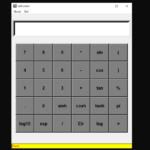## Scientific Calculator In Python With Source Code

The Scientific Calculator In Python is a simple project developed using Python. The project file contains a python script (my_Calculator.py). This is a simple GUI-base project which is very easy to understand and use. Also, this project makes a convenient...

Type : Project

File Size : 2.7 KB# Similar Projects and Reports

#### Scientific Calculator In Python With Source Code

The Scientific Calculator In Python is a simple project developed using Python. The project file contains a python script (my_Calculator.py). This is a simple GUI-base project which is very easy to understand and use. Also, this project makes a convenient...

#### Scientific GUI Calculator In Python With Source Code

The Scientific GUI Calculator In Python is a simple project developed using Python. The project file contains a python script (scientific-cal.py). This is a simple GUI-based project which is very easy to understand and use. Also, this project makes a conv...

#### CALCULATOR IN PYTHON WITH SOURCE CODE

This Calculator project In Python is a simple project developed using Python. The project contains the numbers, operators, and sign like in normal calculator. So, the user can enter any number they want in the calculations. Hence, the user can use a simpl...

#### Tip Calculator In Python with Source Code

The Tip Calculator In Python is a simple project developed using Python. The project is for the user convenient, for calculating the tip. This project is an interesting project. The user can calculate the tip amount that they can get from a single bill pa...

#### BMI Calculator In Python With Source Code

The BMI Calculator In Python is a simple project developed using Python. The project is for the user convenient, for checking your BMI. With the BMI value, you can check whether you have a healthy weight or not. The project file contains a python script ...

#### Calculator App In Python With Source Code

The Calculator App In Python is a simple project developed using Python. The project contains the numbers, operators, and signs like on a normal calculator. So, the user cannot enter the number themselves, they can just click on the numbers they want in t...